# 系列之4-单入单出的一层神经网络能做什

$y=a_0+a_1x_1+a_2x_2+\dots+a_kx_k$

# 创造训练数据

import numpy as np
import matplotlib.pyplot as plt
from pathlib import Path

def create_sample_data(m):
# check if saved before
Xfile = Path("XData.npy")
Yfile = Path("YData.npy")
# load data from file
if Xfile.exists() & Yfile.exists():
else: # generate new data
X = np.random.random(m)
# create some offset as noise to simulate real data
noise = np.random.normal(0,0.1,X.shape)
# genarate Y data
W = 2
B = 3
Y = X * W + B + noise
np.save("XData.npy", X)
np.save("YData.npy", Y)
return X, Y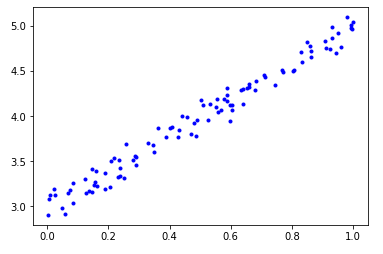$\begin{pmatrix} x_1\\ x_2\\ \dots\\ x_m\\ \end{pmatrix} , \begin{pmatrix} y_1\\ y_2\\ \dots\\ y_m\\ \end{pmatrix}$

### 最小二乘法与均方差

$Error = \frac{1}{m}\sum_{i=1}^m(z(x_i)-y_i)^2 = \frac{1}{m}\sum_{i=1}^m(y_i-wx_i-b)^2$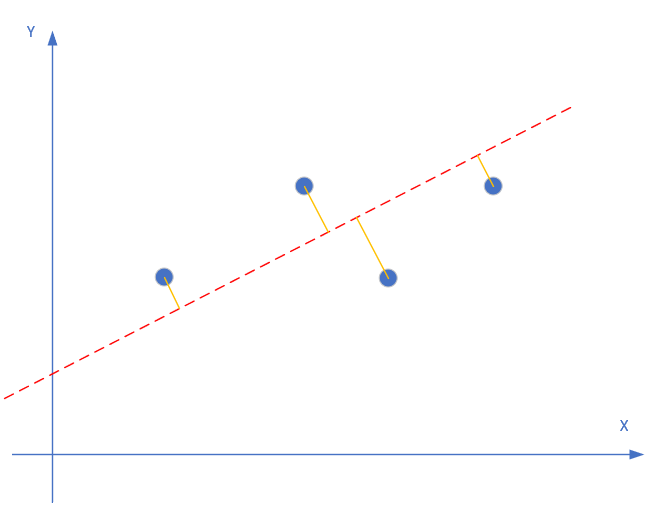$w = \frac{\sum{y_i(x_i-\bar{x})}}{\sum{x_i^2}-\frac{1}{m}(\sum{x_i})^2}\tag{求和均为i=1到m}$
$b=\frac{1}{m}\sum_{i=1}^m(y_i-wx_i)$

x_sum = sum(X)                          # 求x之和
x_mean = x_sum/m                        # 求x平均
x_square = sum(X*X)                     # 求x平方之和
x_square_mean = x_sum * x_sum / m       # 求x之和之平方之均
xy = sum(Y*(X-x_mean))                  # 求w的公式的分子部分
w = xy / (x_square - x_square_mean)     # 求w
print(w)
b = sum(Y-w*X) / m                      # 求b
print(b)

w=1.9983541668129974
b=3.0128994960012876

$y=a_0+a_1x+a_2x^2+ \dots + a_mx^m \tag{单元x多次方程}$
$y=a_0+a_1x_1+a_2x_2+ \dots + a_mx_m \tag{多元x线性方程}$

$y=0.4x^2 + 0.3xsin(15x) + 0.01cos(50x)-0.3$
$y=3x_1^2 + 4x_2$

# 定义神经网络结构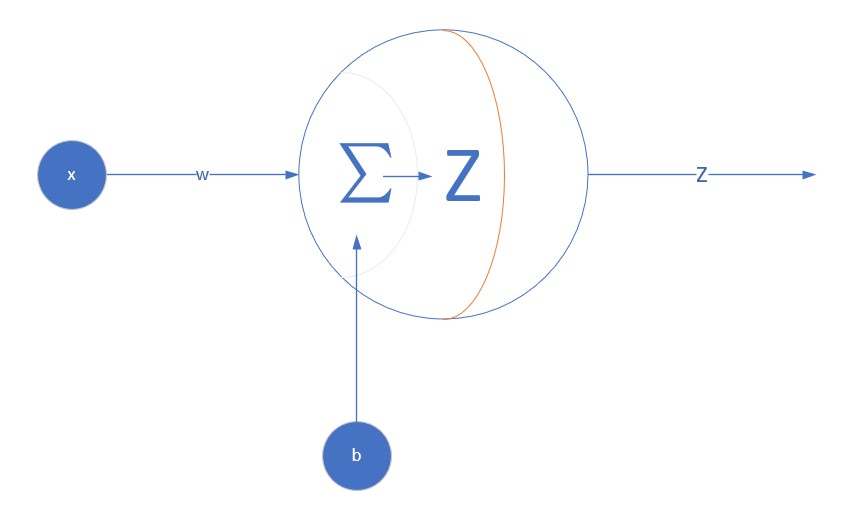def forward_calculation(w,b,X):
z = w * x + b
return z

# 定义代价函数

$loss = \frac{1}{2m}\sum_{i=1}^{m}(Z_i - Y_i) ^ 2$

# w:weight, b:bias, X,Y:sample data, count: count of sample, prev_loss:last time's loss
def check_diff(w, b, X, Y, count, prev_loss):
Z = w * X + b
LOSS = (Z - Y)**2
loss = LOSS.sum()/count/2
diff_loss = abs(loss - prev_loss)
return loss, diff_loss

# 定义针对w和b的梯度函数

## 求w的梯度

$z = wx+b$

$loss = \frac{1}{2}(z-y)^2$

$\frac{\partial{loss}}{\partial{w}} = \frac{\partial{loss}}{\partial{z}}*\frac{\partial{z}}{\partial{w}}$

$\frac{\partial{loss}}{\partial{z}} = \frac{\partial{(\frac{1}{2}(z-y)^2)}}{\partial{z}} = z-y$

$\frac{\partial{z}}{\partial{w}} = \frac{\partial{}}{\partial{w}}(wx+b) = x$

$\frac{\partial{loss}}{\partial{w}} = \frac{\partial{loss}}{\partial{z}}*\frac{\partial{z}}{\partial{w}} = (z-y)x$

## 求b的梯度

$\frac{\partial{loss}}{\partial{b}} = \frac{\partial{loss}}{\partial{z}}*\frac{\partial{z}}{\partial{b}}$

$\frac{\partial{z}}{\partial{b}} = \frac{\partial{(wx+b)}}{\partial{b}} = 1$

$\frac{\partial{loss}}{\partial{b}} = \frac{\partial{loss}}{\partial{z}}*\frac{\partial{z}}{\partial{b}} = z-y$

# z:predication value, y:sample data label, x:sample data, count:count of sample data
def dJwb_batch(X,Y,Z,count):
p = Z - Y
db = sum(p)/count
q = p * X
dw = sum(q)/count
return dw, db

def dJwb_single(x,y,z):
p = z - y
db = p
dw = p * x
return dw, db


# 每次迭代后更新w,b的值

def update_weights(w, b, dw, db, eta):
w = w - eta*dw
b = b - eta*db
return w,b

# 帮助函数

def show_result(X, Y, w, b, iteration, loss_his, w_his, b_his, n):
# draw sample data
#    plt.figure(1)
plt.subplot(121)
plt.plot(X, Y, "b.")
# draw predication data
Z = w*X +b
plt.plot(X, Z, "r")
plt.subplot(122)
plt.plot(loss_his[0:n], "r")
plt.plot(w_his[0:n], "b")
plt.plot(b_his[0:n], "g")
plt.grid(True)
plt.show()
print(iteration)
print(w,b)

def print_progress(iteration, loss, diff_loss, w, b, loss_his, w_his, b_his):
if iteration % 10 == 0:
print(iteration, diff_loss, w, b)
loss_his = np.append(loss_his, loss)
w_his = np.append(w_his, w)
b_his = np.append(b_his, b)
return loss_his, w_his, b_his

# 主程序初始化

# count of samples
m = 200
# initialize_data
eta = 0.01
# set w,b=0, you can set to others values to have a try
w, b = 0, 0
eps = 1e-10
iteration, max_iteration = 0, 10000
# calculate loss to decide the stop condition
prev_loss, loss, diff_loss = 0,0,0
# create mock up data
X, Y = create_sample_data(m)
# create list history
loss_his, w_his, b_his = list(), list(), list()

# 训练方式的选择

1. 随机梯度下降SGD (Stochastic Gradient Descent)：每次迭代只使用一个样本进行训练，每次都更新梯度值
2. 批量梯度下降BGD (Batch Gradient Descent)：把所有样本整批的输入网络进行训练，每批样本更新一次梯度值
3. 小批量梯度下降MBGD (Mini-batch Gradient Descent)：每次用一部分样本进行训练，每小批样本更新一次梯度值

Pseudo code如下：

repeat:
for 每个样本x,y:
标量计算得到z的单值 z = w * x + b
计算w的梯度
计算b的梯度
更新w,b的值
计算本次损失
与上一次的损失值比较，足够小的话就停止训练
end for
until stop condition

repeat:
矩阵前向计算得到Z值 = w * X + b（其中X是所有样本的数组）
计算w的梯度
计算b的梯度
更新w,b的值
计算本批损失
与上一批的损失值比较，足够小的话就停止训练
until stop condition

repeat:
从样本集X中获得一小批量样本Xn
矩阵前向计算得到Z值 = w * Xn + b（其中Xn是一小批样本的数组）
计算w的梯度
计算b的梯度
更新w,b的值
计算本批损失
与上一批的损失值比较，足够小的话就停止训练
until stop condition

## 随机梯度下降方式 - SGD

### 程序主循环

while iteration < max_iteration:
for i in range(m):
# get x and y value for one sample
x = X[i]
y = Y[i]
# get z from x,y
z = forward_calculation(w, b, x)
# calculate gradient of w and b
dw, db = dJwb_single(x, y, z)
# update w,b
w, b = update_weights(w, b, dw, db, eta)
# calculate loss for this batch
loss, diff_loss = check_diff(w,b,X,Y,m,prev_loss)
# condition 1 to stop
if diff_loss < eps:
break
prev_loss = loss

iteration += 1
loss_his, w_his, b_his = print_progress(iteration, loss, diff_loss, w, b, loss_his, w_his, b_his)
if diff_loss < eps:
break

show_result(X, Y, w, b, iteration, loss_his, w_his, b_his, 200)

### 程序运行结果

1 0.0013946089980010831 1.7082689753500857 2.8635473444149815
2 1.2964547916170625e-05 1.8540100768184453 3.06775776515801
3 7.79019593934345e-07 1.8807160337440225 3.0745103188170186
......
19 8.734980997196495e-09 1.9871421670235265 3.0189893623564035
20 6.770768725197773e-09 1.9888753203686051 3.0180574393623383
21 1.4217967081453509e-13 1.9909568305589769 3.0231282539481192
21
1.9909568305589769 3.0231282539481192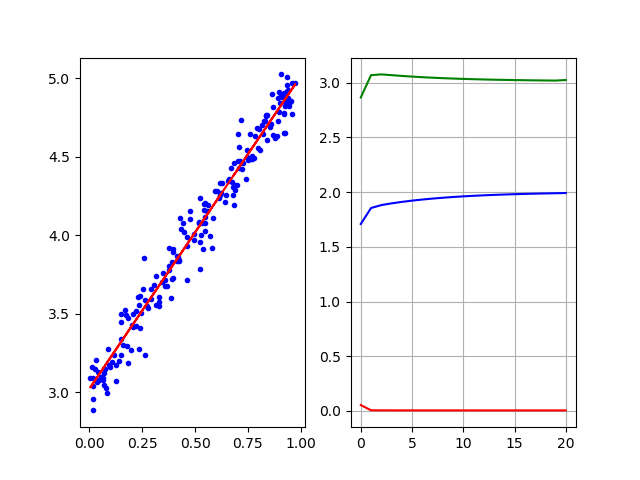## 批量梯度下降方式 - BGD

### 程序主循环

# condition 2 to stop
while iteration < max_iteration:
# using current w,b to calculate Z
Z = forward_calculation(w,b,X)
# get gradient value
dW, dB = dJwb_batch(X, Y, Z, m)
# update w and b
w, b = update_weights(w, b, dW, dB, eta)
#   print(iteration,w,b)
iteration += 1
# condition 1 to stop
loss, diff_loss = check_diff(w,b,X,Y,m,prev_loss)
if diff_loss < eps:
break
prev_loss = loss
iteration += 1
loss_his, w_his, b_his = print_progress(iteration, loss, diff_loss, w, b, loss_his, w_his, b_his)

show_result(X, Y, w, b, iteration, loss_his, w_his, b_his, 200)

### 程序运行结果

15580 1.0078619969849933e-10 1.9970527059142416 3.013622182124774
15590 1.0010891421385892e-10 1.9970570862183055 3.0136197497927952
15591
1.9970579605084025 3.013619264309657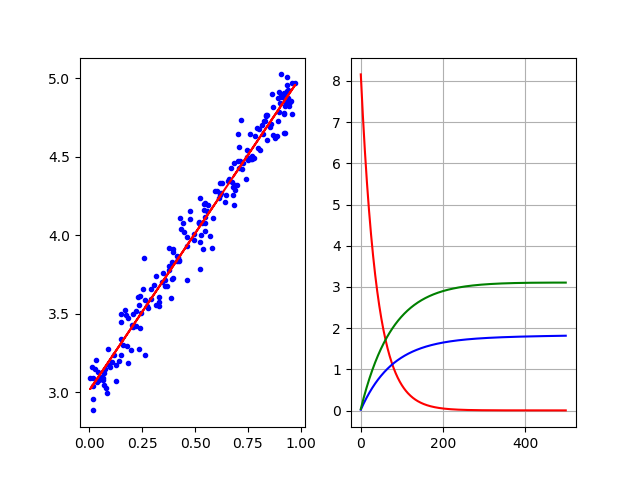## 小批量梯度下降方式 - MBGD

### 程序主循环

batchNumber = 20 # 设置每批的数据量为20

# condition 2 to stop
while iteration < max_iteration:
# generate current batch
batchX, batchY = generate_batch(X, Y, iteration, batchNumber, m)
# using current w,b to calculate Z
Z = forward_calculation(w,b,batchX)
# get gradient value
dW, dB = dJwb_batch(batchX, batchY, Z, batchNumber)
# update w and b
w, b = update_weights(w, b, dW, dB, eta)
# calculate loss
loss, diff_loss = check_diff(w,b,X,Y,m,prev_loss)
# condition 1 to stop
if diff_loss < eps:
break
prev_loss = loss
iteration += 1

loss_his, w_his, b_his = print_progress(iteration, loss, diff_loss, w, b, loss_his, w_his, b_his)

show_result(X, Y, w, b, iteration, loss_his, w_his, b_his, 300)

### 程序运行结果

4450 1.2225522157127688e-10 1.9753608229361126 3.0087345373193264
4460 1.1451271614976166e-10 1.9753871799671467 3.008717691199392
4470 1.068962461950318e-10 1.975413384400498 3.0087009426124025
4479
1.975439437119652 3.0086842909936786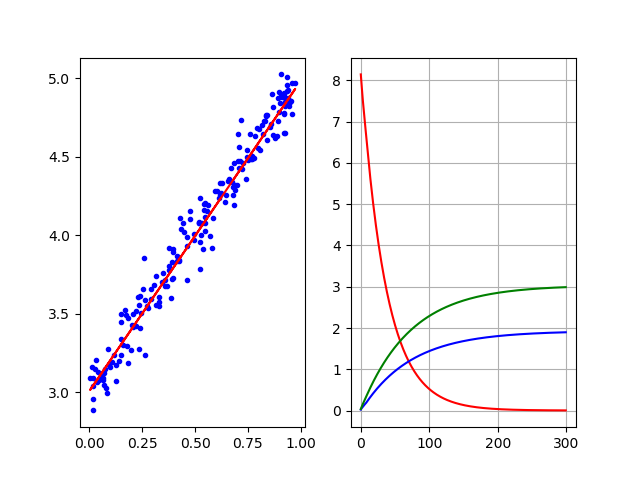# 三种方式的比较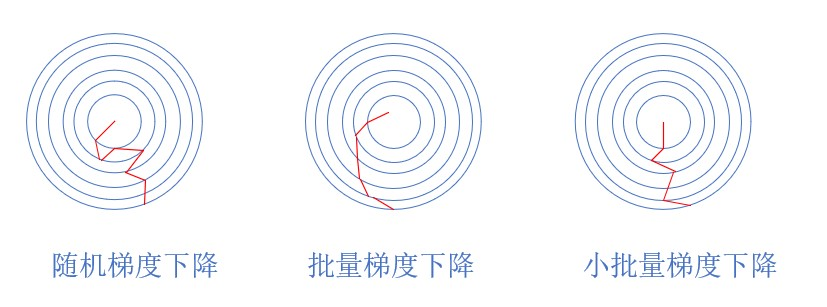## 随机梯度下降

1. 每次用一个样本训练，然后立刻更新权重，训练速度最快。可以简单地理解为“神经过敏”性格。
2. 可以设置一个适中（更多）的迭代次数，以便得到更好的解
3. 由于使用单个样本数据，会受数据噪音的影响，且前后两个样本的训练效果可能会相互抵消。从轨迹上来看，跳跃性较大。
4. 由于数据随机，所以有可能受训练样本噪音影响而跳到全局最优解，但是不保证。在某些博客中说“本方法只能获得局部最优解”，这实际上是不对的。

## 批量梯度下降

1. 每次用整批样本训练后，才更新一次权重，训练速度最慢。可以简单地理解为“老成持重”性格。
2. 特定的样本如果误差较大，不会影响整体的训练质量
3. 从轨迹上来看，比较平稳地接近中心，但是在接近最优解时的迭代次数太多，非常缓慢
4. 如果只有一个极小值，可以得到相对全局较优的解。如果实际数据有两个极小值，不一定能得到全局最优解。在某些博客中说“本方法可以获得全局最优解”，这实际上是不能保证的，取决于初始值设置。

## 小批量梯度下降

1. 每次使用一小批数据训练，速度适中。可以简单地理解为“稳重而不失灵活”的性格。
2. 多了一个batchNumber参数需要设置，大家可以试验一下分别设置为10，20，25，40，50，训练效果如何
3. 从轨迹上来看，有小的跳跃，但是下降方向还算是稳定，不会向反方向跳跃posted @ 2018-11-20 14:02 UniversalAIPlatform 阅读(...) 评论(...) 编辑 收藏